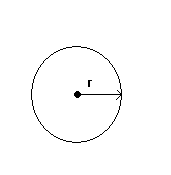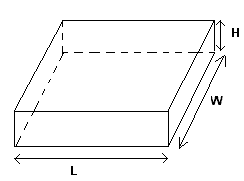# Table of Formulas For Geometry

A table of formulas for geometry, related to area and perimeter of triangles, rectangles, cercles, sectors, and volume of sphere, cone, cylinder are presented.

## Right Triangle and Pythagora's theorem

Pythagora's theorem: The two sides a and b of a right triangle and the hypotenuse c are related by

a 2 + b 2 = c 2## Area and Perimeter of TrianglePerimeter = a + b + c

There are several formulas for the area.

If the base b and the corresponding height h are known, we use the formula

Area = (1 / 2) � b � h.

If two sides and the angle between them are known, we use one of the formulas, depending on which side and which angle are known

Area = (1 / 2) � b � c sin A

Area = (1 / 2) � a � c sin B

Area = (1 / 2) � a � b sin C .

If all three sides are known, we may use Heron's formula for the area.

Area = √ [ s(s - a)(s - b)(s - c) ] , where s = (a + b + c)/2.

## Area and Perimeter of RectanglePerimeter = 2L + 2W
Area = L � W

## Area of ParallelogramArea = b � h

## Area of TrapezoidArea = (1 / 2)(a + b) � h

## Circumference of a Circle and Area of a Circular RegionCircumference = 2 � π � r
Area = π � r 2

## Arclength and Area of a Circular SectorArclength: s = r � t

Area = (1/2) � r 2 � t

where t is the central angle in

## Volume and Surface Area of a Rectangular SolidVolume = L*W*H
Surface Area = 2(L � W + H � W + H � L)

## Volume and Surface Area of a SphereVolume = (4/3) � π � r 3
Surface Area = 4 � π � r 2

## Volume and Surface Area of a Right Circular CylinderVolume = π � r 2 � h
Total Surface Area = 2 � π � r � h + 2 � π � r 2

## Volume and Surface Area of a Right Circular ConeVolume = (1/3) � π � r 2 � h
Surface Area = π � r � √ (r 2 + h 2)